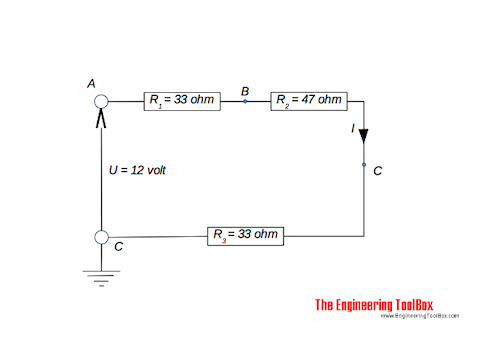Engineering ToolBox - Resources, Tools and Basic Information for Engineering and Design of Technical Applications!

# Relative vs. Absolute Voltage

## Electric circuits and voltage at any point.### Absolute Voltage

If voltage at point A is quoted with reference to the earth point - the absolute potential - then the voltage is written UA.

### Relative Voltage

If voltage at point A is quoted with reference to point B - then the voltage is written UAB.

### Example - Relative and Absolute Voltage

The total resistance in the series circuit above can be calculated

R = (33 ohm) + (47 ohm) + (33 ohm)

= 113 ohm

The current in the circuit can be calculated

I = (12 volts) / (113 ohm)

= 0.11 ampere

The relative voltage between point A and BVAB - can be calculated

UAB = (33 ohm) (0.11 ampere)

= 3.6 volts

The absolute voltage in point A relative to earth is

UA = 12 V

The absolute voltage in point B relative to earth is

UB = UA - UAB

= (12 volt) - (3.6 volt)

= 8.4 volt

## Related Topics

• ### Electrical

Electrical units, amps and electrical wiring, wire gauge and AWG, electrical formulas and motors.

## Related Documents

• ### Current Divider - Online Calculator

An electric current divider outputs a current that is a fraction of the input current.
• ### Electric Circuit Diagram - Drawing Template

Online shareable electric circuit diagram.
• ### Electric Circuits Voltage Drop

Ohm's law and voltage drop in electric circuits.
• ### Electrical Formulas

Commonly used electrical formulas like Ohms Law and more.
• ### Electrical Series Circuits

Voltage and current in electrical series circuits.
• ### Kirchhoff's Voltage and Current Laws

Kirchhoff's current and voltage laws.

## Engineering ToolBox - SketchUp Extension - Online 3D modeling!

Add standard and customized parametric components - like flange beams, lumbers, piping, stairs and more - to your Sketchup model with the Engineering ToolBox - SketchUp Extension - enabled for use with older versions of the amazing SketchUp Make and the newer "up to date" SketchUp Pro . Add the Engineering ToolBox extension to your SketchUp Make/Pro from the Extension Warehouse !

We don't collect information from our users. More about

## Citation

• The Engineering ToolBox (2016). Relative vs. Absolute Voltage. [online] Available at: https://www.engineeringtoolbox.com/relative-absolute-voltage-d_1922.html [Accessed Day Month Year].

Modify the access date according your visit.

9.29.12